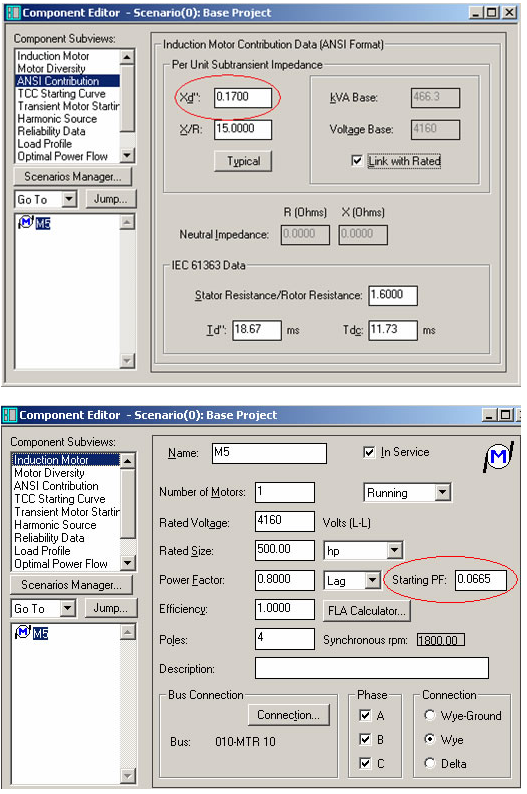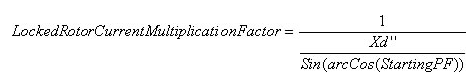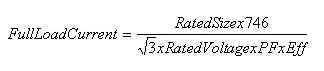• Sales
• Library Model Request
• Technical Support

# How is the induction motor startup current multiplication factor calculated?

The startup current multiplication factor in load-flow is calculated from the inverse of the motor Subtransient Reactance and the Starting Power Factor. The reactance of this impedance (Xd”) can be found in the Component Editor under the ANSI/IEC Contribution subview but the Starting Power (StartingPF) Factor needs to be read from the Induction Motor Subview.The applicable equation is:This Locked Rotor Current Multiplication Factor is multiplied by the Full Load Nominal Current of the motor. The Full Load Nominal Current is calculated from the motor rated values defined in the Induction motor subview window.where:
RatedSize is the Rated Size of motor in HP
RatedVoltage is the Rated Voltage of the motor in Volts
PF is the Power Factor of the motor in p.u.
Eff is the Efficiency of the motor in p.u.